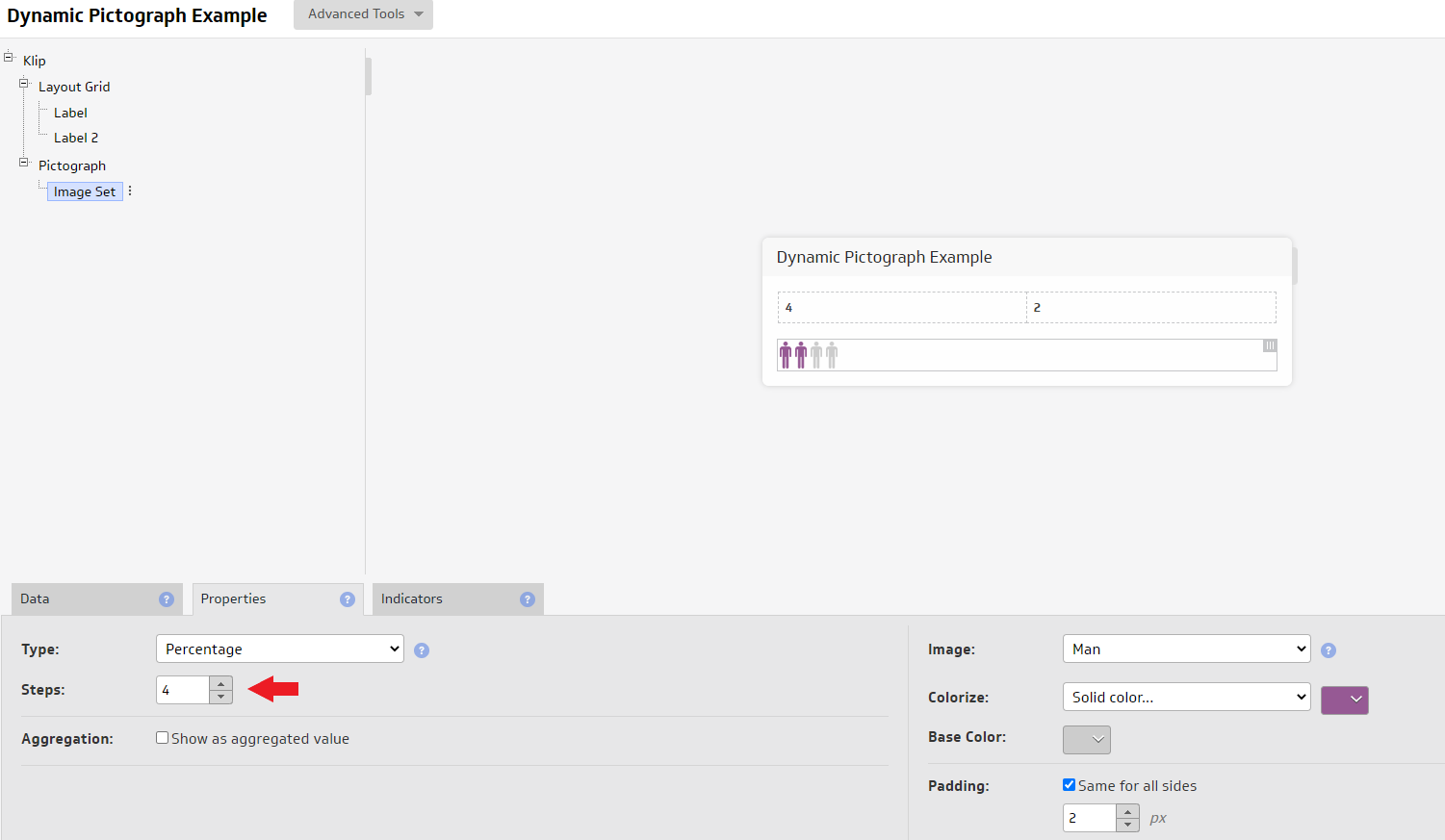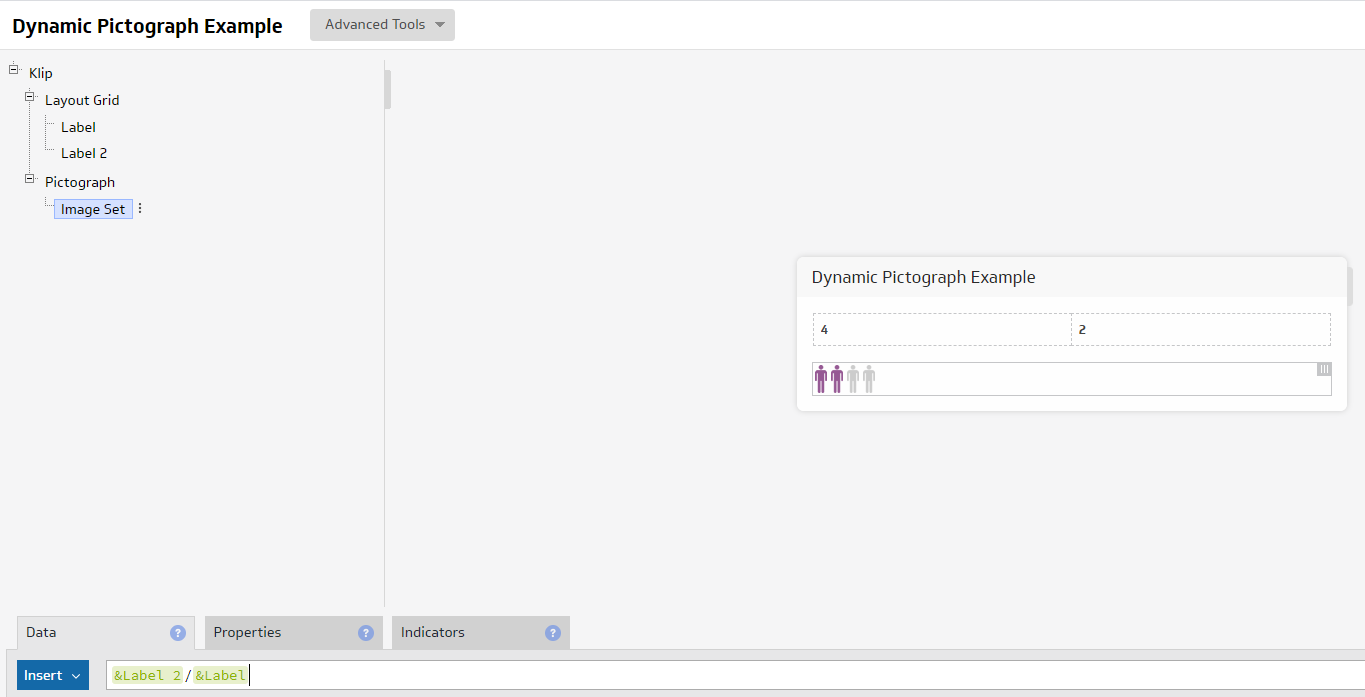Currently the number of steps in a pictograph is a fixed number. Would be nice to be able to change that with a function or formula.

Example where I would use this:

a) Number of 'persons' that start the process (value of steps in pictograph)
b) Percentage of 'persons' that completed the process. (data field: completed/started)

•Meggan King

Hi Peter,

This should be possible. If your Properties > Increment is set to 1, then your DATA can be a calculation. The result of that calculation will be how many times the picture icon is displayed. So if your initial value calculates to 5, then the result will be 5 pictures, if the calculation changes - due to your data changing in the data source, a variable selected, etc, then it would increase or decrease. You can do any formulas in the DATA field which you normally would use to run your calculation in Klipfolio.

You can read more here: https://support.klipfolio.com/hc/en-us/articles/216181037-How-to-build-Pictograph-components

Thanks

Meggan

•Peter Vermeulen

Hi Megan,

I want to be able to make the number of steps to change dynamically as the number in your label above changes, while the formula in the data remains the percentage calculation of the results of the second label out of the results of the first label.

I want to add a function or formula here:While keeping the data formula a calculation as in example here:Regards,
Peter